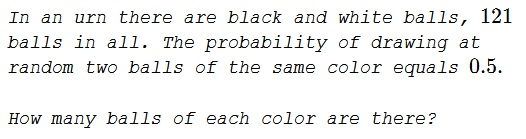# Two Balls of the Same Color

### Problem### Solution 1

Let there be $b$ black and $w$ white balls. We have $b+w=121$ and

\displaystyle\begin{align}\frac{1}{2}&=\frac{w}{121}\cdot\frac{w-1}{120}+\frac{b}{121}\cdot\frac{b-1}{120}\\ &=\frac{w(w-1)+b(b-1)}{121\cdot 120}. \end{align}

It follows that $b$ and $w$ satisfy anther equation

$\displaystyle 121\cdot 60=w^2+b^2-(w+b)=w^2+b^2-121,$

so that $w^2+b^2=121\cdot 61.$ Now, $(w+b)^2=121^2$ from which $wb=121\cdot 30.$ Thus $w$ and $b$ are the roots of the quadratic equation

$x^2-121x+121\cdot 30.$

Applying the quadratic formula

\displaystyle\begin{align} x_{1,2}&=\frac{121\pm\sqrt{121^2-4\cdot 121\cdot 30}}{2}\\ &=\frac{121\pm\sqrt{121(121-120}}{2}=\frac{121\pm 11}{2}. \end{align}

Then, e.g., $x_1=55$ and $x_2=66$ which could be the numbers of black and white balls, or vice versa.

$55$ and $66$ are successive triangular number and sum up to $121,$ a square.

### Solution 2

If we do that in general

\displaystyle\begin{align}\frac{1}{2}&=\frac{w}{w+b}\cdot\frac{w-1}{w+b-1}+\frac{b}{w+b}\cdot\frac{b-1}{w+b-1}\\ &=\frac{w(w-1)+b(b-1)}{(w+b)\cdot (w+b-1)}. \end{align}

and multiply out, we get

$(w+b)(w+b-1)=2w(w-1)+2b(b-1)=2w^2+2b^2-2\cdot (w+b),$

or

$(w-b)^2=w+b=121,$

meaning that, assuming $w\ge b,$ $w-b=11.$ Combining this with $w+b=121$ we get the same result as above.

### Solution 3

We have four possible outcomes:

$S=\left(\begin{array}{CC}W&W\\W&B\\B&W\\B&B\end{array}\right).$

We have

\displaystyle \begin{align} P(WW || BB)&=\frac{W}{121}\cdot\frac{W-1}{120}+\frac{B}{121}\cdot\frac{B-1}{120}=\frac{1}{2}\\ P(WB || BW)&=\frac{W}{121}\cdot\frac{B}{120}+\frac{B}{121}\cdot\frac{W}{120}=\frac{1}{2}. \end{align}

Solving we get $B^2+W^2=7260+b+W$ and $BW=3630$ from which one is $66,$ the other $55.$

### Acknowledgment

This is a paraphrase of problem 65284 from a Russian problem collection.

Solution 3 is by N. N. Taleb.Copyright © 1996-2018 Alexander Bogomolny

 65278418